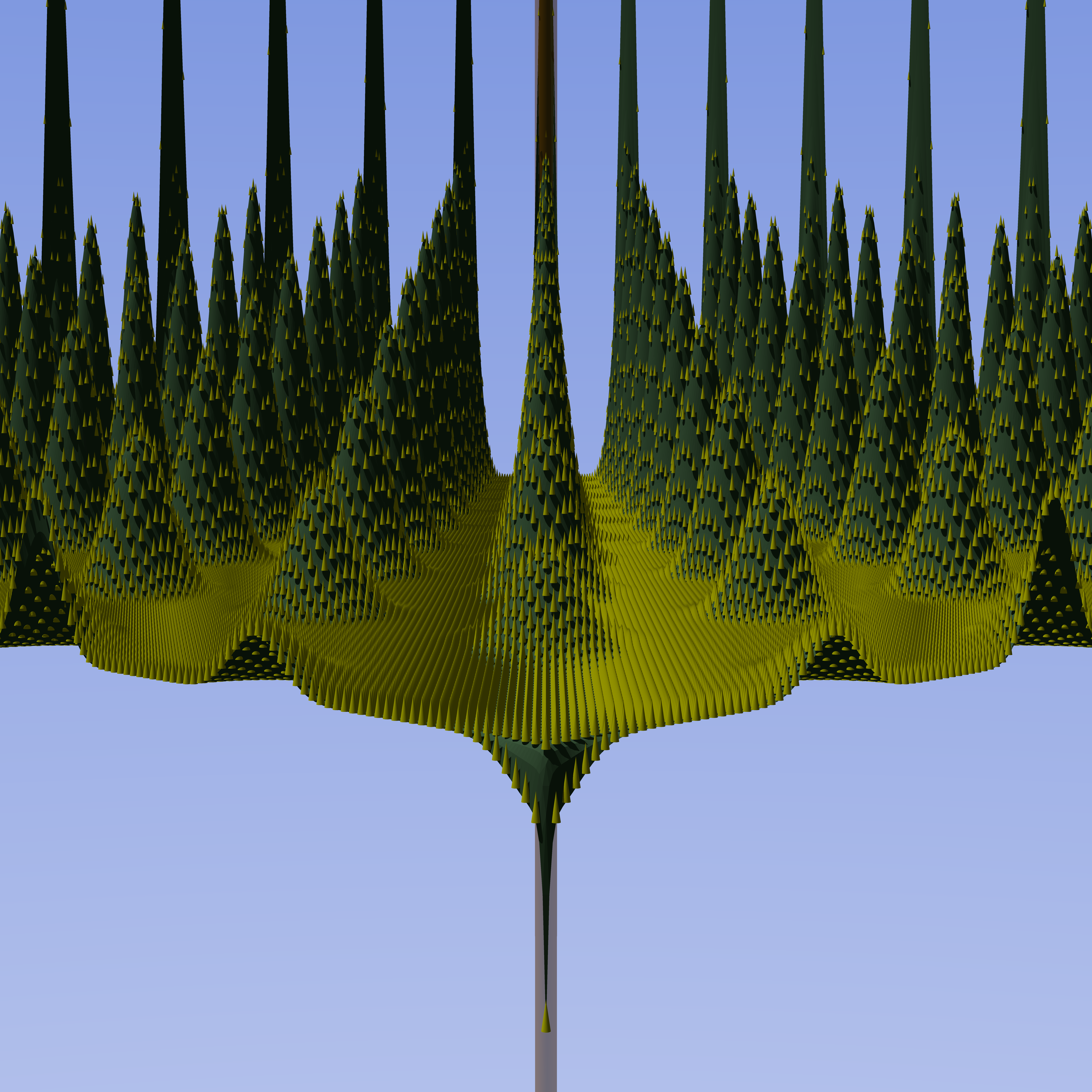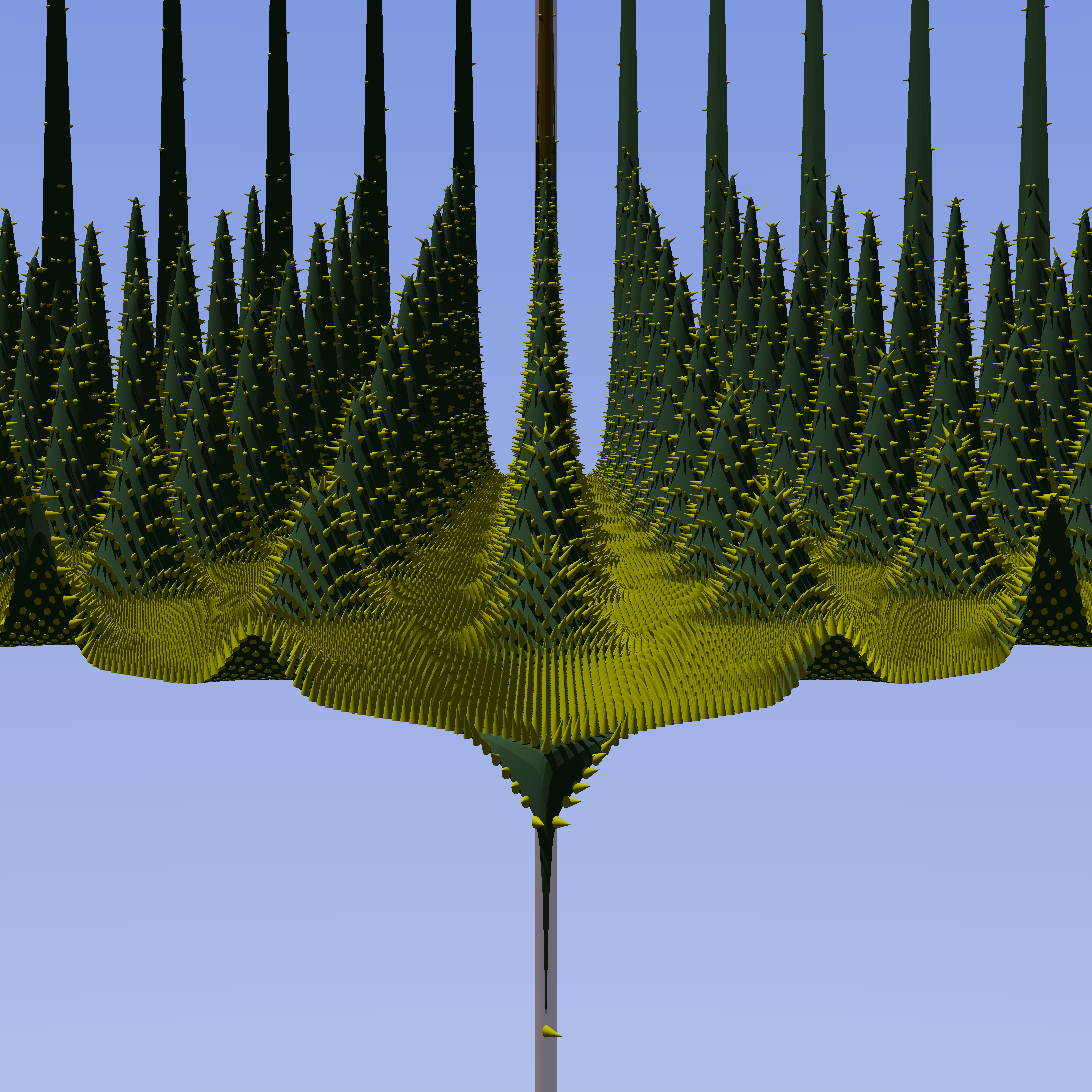## How to place objects perpendicular to surface or what is a normal vector (in povray)

I want to place objects perpendicular to a (curved) surface, this surface is defined as a square matrix where value denotes height of the surface at a given point. To illustrate, let's I want spikes in the below image to be perpendicular to the surface:I want spikes in the below image to be perpendicular to the surface.

In practice curved survace I want to place object's on is povray height_field object. Here is height_field documentation.

## Normal vector

Vector that is perpendicular to the surface at a given point is called a "normal vector". Normal vector is closely related to gradient.

My surface is defined as:

\begin{equation*} y = F(x, z) \end{equation*}

where F(x, z) is "the value of matrix at point (x, z)". (Povray uses slightly non-standard coordinate system, explained here).

So wikipedia explains that formula for normal vector $$\vec{n}$$ should be (this is converted into povray coordinate system):

\begin{equation*} \vec{n} = \left[-\frac{\partial y}{\partial x}, 1, -\frac{\partial y}{\partial z}\right] \end{equation*}

I use numpy.gradient function to calculate the gradient, however preparing the input array is non trivial.

Input for the agorithm is a 2d matrix in a format that is easily exportable to povray. To render the matrix I use height_field element, which loads a gray-scale png image, and the lighter the dot is the higher this element of height field is. So the input data is a square 2d matrix containing unsigned 16bit ints.

Height field then has a bounding box from $$(0, 0, 0)$$ to $$(1, 1, 1)$$, and then can be normally translated or scaled. So far I support only scaling along the y axis (which is height for povray).

So to prepare the input array you need to:

1. Convert it into float array;
2. Scale it so values are from 0 to 1 (or if your height field is scaled you need to scale it to appropriate value).
3. Multiply values in the array by number of elements along one of the axes (I assume matrix is square). np.gradient assumes that distance between elements of the array is a 1 unit, however distance between points of the height_field for povray is 1 / number of pixels.

So to calculate gradient you need following code:

def scale_stage(stage: np.ndarray, vertical_scale: float) -> np.ndarray:
assert stage.shape == stage.shape
stage = np.asarray(stage, dtype=float)
stage -= np.min(stage)
stage /= np.max(stage)
stage *= vertical_scale
return stage

"""
:param stage: Input for height_field element
:param vertical_scale: Height of the resulting height field in povray units.
:return:
"""

stage = scale_stage(stage, vertical_scale)

stage *= stage.shape

# This is numpy weirdness.


## Rotate object to be perpendicular to normal vector

I assume that object is oriented along the $$[0, 1, 0]$$ vector, i.e. it is facing upwards. I want to rotate this object so it is parallel to normal vector $$\vec{n} = \left[x, 1, z\right]$$, to do this I need to obtain coefficients of rotation vector $$\vec{rot}= \left[\alpha, \beta, \gamma\right]$$.

Some trigonometry (that is not that hard, but at the same time would be tedious to explain) tells me that:

\begin{equation*} \vec{n} = \left[x, 1, z\right] = \left[-\frac{\partial y}{\partial x}, 1, -\frac{\partial y}{\partial z}\right] \end{equation*}
\begin{equation*} \vec{rot} = \left[\arctan(x), 0, -arctan(z)\right] = \left[-\arctan\left(\frac{\partial y}{\partial x}\right), 0, \arctan\left(\frac{\partial y}{\partial z}\right)\right] \end{equation*}

Please note that that z axis is negated, this is due povray using weird coordinate system.

## Final code

Last touch is converting results of arcus tangens from radians (used in numpy) to degress (used in povray):

def compute_rotation_angles(stage: np.ndarray, vertical_scale: float):
new_shape = (stage.shape, stage.shape, 3)
angles = np.zeros(new_shape, dtype=np.float64)
angles[:, :, 0] = - (np.arctan(gradx) / math.pi * 180)
angles[:, :, 2] = (np.arctan(gradz) / math.pi * 180)
return angles


## Note on rotating objects

Povray always rotates objects relative to the axes of the coordinate system.

So to rotate it aloing it's "center" you'll need to:

1. Place it initially at the center of the coordinate system;
2. Rotate it;
3. Translate it into appropriate position.

So for example to have cone rotated by $$30\deg$$ along the x axis placed at $$[1, 1, 1]$$, you'll need to:

    // rotate the object first
rotate <30, 0, 0>
// then translate it where it belongs
translate <1, 1, 1>
}


## Finished resultsFinished results.